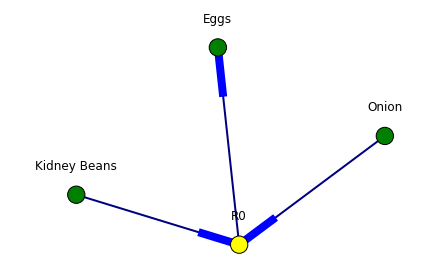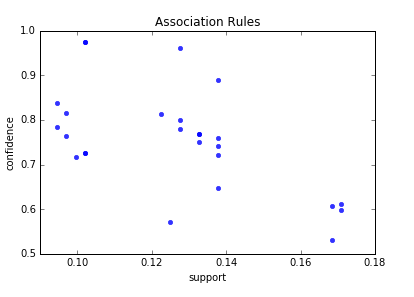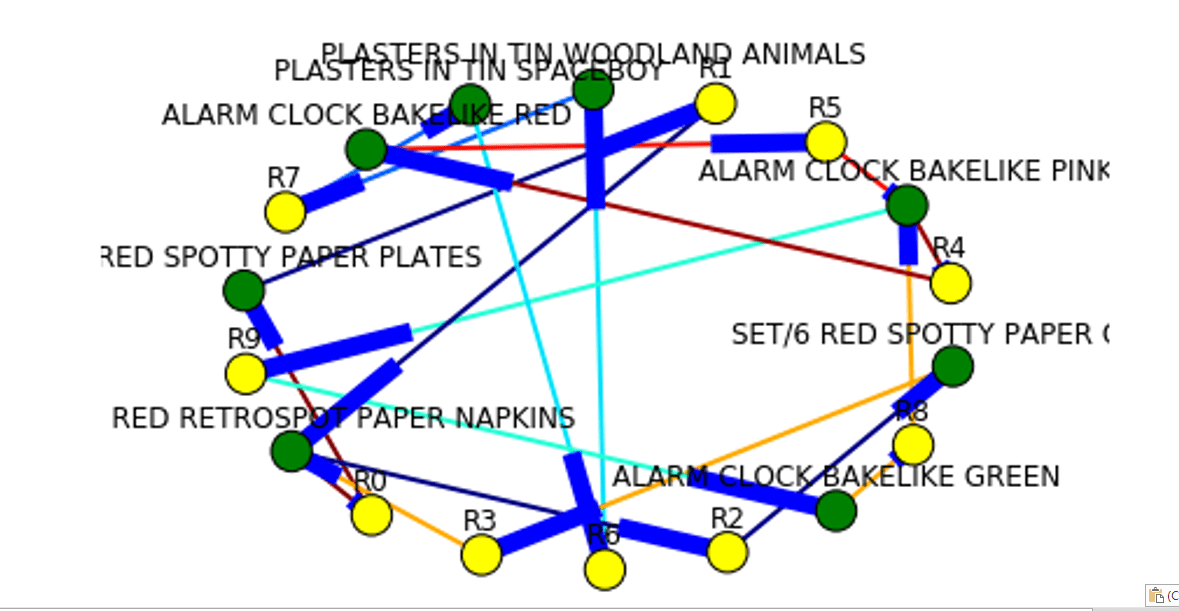## Time Series Prediction with LSTM and Keras for Multiple Steps Ahead

In this post I will share experiment with Time Series Prediction with LSTM and Keras. LSTM neural network is used in this experiment for multiple steps ahead for stock prices data. The experiment is based on the paper . The authors of the paper examine independent value prediction approach. With this approach a separate model is built for each prediction step. This approach helps to avoid error accumulation problem that we have when we use multi-stage step prediction.

### LSTM Implementation

Following this approach I decided to use Long Short-Term Memory network or LSTM network for daily data stock price prediction. LSTM is a type of recurrent neural network used in deep learning. LSTMs have been used to advance the state-of the-art for many difficult problems. 

For this time series prediction I selected the number of steps to predict ahead = 3 and built 3 LSTM models with Keras in python. For each model I used different variable (fit0, fit1, fit2) to avoid any “memory leakage” between models.
The model initialization code is the same for all 3 models except changing parameters (number of neurons in LSTM layer)
The architecture of the system is shown on the fig below.

Here we have 3 LSTM models that are getting same X input data but different target Y data. The target data is shifted by number of steps. If model is forecasting the data stock price for day 2 then Y is shifted by 2 elements.
This happens in the following line when i=1:

```yt_ = yt.shift (-i - 1  )
```

The data were obtained from stock prices from Internet.

The number of unit was obtained by running several variations and chosen based on MSE as following:

```
if i==0:
units=20
batch_size=1
if i==1:
units=15
batch_size=1
if i==2:
units=80
batch_size=1
```

If you want run more than 3 steps / models you will need to add parameters to the above code. Additionally you will need add model initialization code shown below.

Each LSTM network was constructed as following:

```
if i == 0 :
fit0 = Sequential ()
fit0.add (LSTM (  units , activation = 'tanh', inner_activation = 'hard_sigmoid' , input_shape =(len(cols), 1) ))
fit0.add (Dense (output_dim =1, activation = 'linear'))
fit0.compile (loss ="mean_squared_error" , optimizer = "adam")

fit0.fit (x_train, y_train, batch_size =batch_size, nb_epoch =25, shuffle = False)
train_mse[i] = fit0.evaluate (x_train, y_train, batch_size =batch_size)
test_mse[i] = fit0.evaluate (x_test, y_test, batch_size =batch_size)
pred = fit0.predict (x_test)
pred = scaler_y.inverse_transform (np. array (pred). reshape ((len( pred), 1)))
# below is just fo i == 0
for j in range (len(pred)) :
prediction_data[j] = pred[j]
```

For each model the code is saving last forecasted number.
Additionally at step i=0 predicted data is saved for comparison with actual data:

```prediction_data = np.asarray(prediction_data)
prediction_data = prediction_data.ravel()

# shift back by one step
for j in range (len(prediction_data) - 1 ):
prediction_data[len(prediction_data) - j - 1  ] =  prediction_data[len(prediction_data) - 1 - j - 1]

# combine prediction data from first model and last predicted data from each model
prediction_data = np.append(prediction_data, forecast)
```

The full python source code for time series prediction with LSTM in python is shown here

Data can be found here

### Experiment Results

The LSTM neural network was running with the following performance:

```train_mse
[0.01846262458218137, 0.009637593373373323, 0.0018845983509225203]
test_mse
[0.01648362025879952, 0.026161141224167357, 0.01774421124347165]
```

Below is the graph of actual data vs data testing data, including last 3 stock data prices from each model.

Accuracy of prediction 98% calculated for last 3 data stock prices (one from each model).

The experiment confirmed that using models (one model for each step) in multistep-ahead time series prediction has advantages. With this method we can adjust parameters of needed LSTM for each step. For example, number of neurons for i=2 was modified to decrease prediction error for this step. And it did not affect predictions for other steps. This is one of machine learning techniques for stock prediction that is described in 

## Prediction on Next Stock Market Correction

On Feb. 6, 2018, the stock market officially entered “correction” territory. A stock market correction is defined as a drop of at least 10% or more for an index or stock from its recent high.  During one week the stock data prices (closed price) were decreasing for many stocks. Are there any signals that can be used to predict next stock market correction?

I pulled historical data from 20 stocks selected randomly and then created python program that counts how many stocks (closed price) were decreased, increased or did not change for each day (comparing with previous day). The numbers then converted into percentage. So if all 20 stock closed prices decreased at some day it would be 100%. For now I was just looking at % of decreased stocks per day. Below is the graph for decreasing stocks. Highlighted zone A is when we many decreasing stocks during the correction.

### Observations

I did not find good strong signal to predict market correction but probably more analysis needed. However before this correction there was some increasing trend for number of stocks that close at lower prices. This is shown below. On this graph the trend line can be viewed as indicator of stock market direction.

```from pandas_datareader import data as pdr
import time

# put below actual symbols as many as you need
symbols=['XXX','XXX', 'XXX', ...... 'XXX']

def get_data (symbol):

path="C:\\Users\\stocks\\"
data.to_csv( path + symbol+".csv")

return data

for symbol in symbols:
get_data(symbol)
time.sleep(7)
```

### Script for Stock Data Analysis

Here is the program that takes downloaded data and counts the number of decreased/increased/same stocks per day. The results are saved in the file and also plotted. Plots are shown after source code below.

And here is the link to the data output from the below program.

```# -*- coding: utf-8 -*-

import os

path="C:\\Users\\stocks\\"
from datetime import datetime
import pandas as pd
import numpy as np

def days_between(d1, d2):
d1 = datetime.strptime(d1, "%Y-%m-%d")
d2 = datetime.strptime(d2, "%Y-%m-%d")
print (d1)
print (d2)
return abs((d2 - d1).days)

i=10000   # index to replace date
j=20      # index for stock symbols
k=5       # other attributes
data = np.zeros((i,j,k))
symbols=[]

count=0

# get index of previous trade day
# because there is no trades on weekend or holidays
# of just subracting 1
def get_previous_ind(row_ind, col_count ):

k=1
print (str(row_ind) + "   " + str(col_count))
while True:
if  data[row_ind-k][col_count] == 1:
return row_ind-k
else:
k=k+1

if k > 1000 :
return -1

dates=["" for i in range(10000) ]
listOfEntries = os.scandir(path)
for entry in  listOfEntries:

if entry.is_file():
print(entry.name)
stock_data = pd.read_csv (str(path) + str(entry.name))
symbols.append (entry.name)

for index, row in stock_data.iterrows():
ind=days_between(row['Date'], "2002-01-01")

dates[ind] = row['Date']
data[ind][count] = 1
data[ind][count] = row['Close']

if (index > 1):
print(entry.name)
prev_ind=get_previous_ind(ind, count)
delta= 1000*(row['Close'] - data[prev_ind][count])
change=0
if (delta > 0) :
change = 1
if (delta < 0) :
change = -1
data[ind][count] = change
data[ind][count] = 1

count=count+1

upchange=[0 for i in range(10000)]
downchange=[0 for i in range(10000)]
zerochange=[0 for i in range(10000)]
datesnew = ["" for i in range(10000) ]
icount=0
for i in range(10000):
total=0
for j in range (count):

if data[i][j] == 1 :
datesnew[icount]=dates[i]
total=total+1
if (data[i][j] ==0):
zerochange[icount]=zerochange[icount]+1
if (data[i][j] ==1):
upchange[icount]=upchange[icount] + 1
if (data[i][j] == - 1):
downchange[icount]=downchange[icount] + 1

if (total != 0) :
upchange[icount]=100* upchange[icount] / total
downchange[icount]=100* downchange[icount] / total
zerochange[icount]=100* zerochange[icount] / total
print (str(upchange[icount]) + "  " +  str(downchange[icount]) + "  " + str(zerochange[icount]))
icount=icount+1

df=pd.DataFrame({'Date':datesnew, 'upchange':upchange, 'downchange':downchange, 'zerochange':zerochange })
print (df)
df.to_csv("changes.csv", encoding='utf-8', index=False)

import matplotlib.pyplot as plt

downchange=downchange[icount-200:icount]
upchange=upchange[icount-200:icount]
zerochange=zerochange[icount-200:icount]

# Two subplots, the axes array is 1-d
f, axarr = plt.subplots(3, sharex=True)
axarr.plot(downchange)
axarr.set_title('downchange')
axarr.plot(upchange)
axarr.set_title('upchange')
axarr.plot(zerochange)
axarr.set_title('zerochange')
plt.show()
```

## How to Create Data Visualization for Association Rules in Data Mining

Association rule learning is used in machine learning for discovering interesting relations between variables. Apriori algorithm is a popular algorithm for association rules mining and extracting frequent itemsets with applications in association rule learning. It has been designed to operate on databases containing transactions, such as purchases by customers of a store (market basket analysis).  Besides market basket analysis this algorithm can be applied to other problems. For example in web user navigation domain we can search for rules like customer who visited web page A and page B also visited page C.

Python sklearn library does not have Apriori algorithm but recently I come across post  where python library MLxtend was used for Market Basket Analysis. MLxtend has modules for different tasks. In this post I will share how to create data visualization for association rules in data mining using MLxtend for getting association rules and NetworkX module for charting the diagram. First we need to get association rules.

### Getting Association Rules from Array Data

To get association rules you can run the following code

```dataset = [['Milk', 'Onion', 'Nutmeg', 'Kidney Beans', 'Eggs', 'Yogurt'],
['Dill', 'Onion', 'Nutmeg', 'Kidney Beans', 'Eggs', 'Yogurt'],
['Milk', 'Apple', 'Kidney Beans', 'Eggs'],
['Milk', 'Unicorn', 'Corn', 'Kidney Beans', 'Yogurt'],
['Corn', 'Onion', 'Onion', 'Kidney Beans', 'Ice cream', 'Eggs']]

import pandas as pd
from mlxtend.preprocessing import OnehotTransactions
from mlxtend.frequent_patterns import apriori

oht = OnehotTransactions()
oht_ary = oht.fit(dataset).transform(dataset)
df = pd.DataFrame(oht_ary, columns=oht.columns_)
print (df)

frequent_itemsets = apriori(df, min_support=0.6, use_colnames=True)
print (frequent_itemsets)

association_rules(frequent_itemsets, metric="confidence", min_threshold=0.7)
rules = association_rules(frequent_itemsets, metric="lift", min_threshold=1.2)
print (rules)

"""
Below is the output
support                     itemsets
0       0.8                       [Eggs]
1       1.0               [Kidney Beans]
2       0.6                       [Milk]
3       0.6                      [Onion]
4       0.6                     [Yogurt]
5       0.8         [Eggs, Kidney Beans]
6       0.6                [Eggs, Onion]
7       0.6         [Kidney Beans, Milk]
8       0.6        [Kidney Beans, Onion]
9       0.6       [Kidney Beans, Yogurt]
10      0.6  [Eggs, Kidney Beans, Onion]

antecedants            consequents  support  confidence  lift
0  (Kidney Beans, Onion)                 (Eggs)      0.6        1.00  1.25
1   (Kidney Beans, Eggs)                (Onion)      0.8        0.75  1.25
2                (Onion)   (Kidney Beans, Eggs)      0.6        1.00  1.25
3                 (Eggs)  (Kidney Beans, Onion)      0.8        0.75  1.25
4                (Onion)                 (Eggs)      0.6        1.00  1.25
5                 (Eggs)                (Onion)      0.8        0.75  1.25

"""
```

### Confidence and Support in Data Mining

To select interesting rules we can use best-known constraints which are a minimum thresholds on confidence and support.
Support is an indication of how frequently the itemset appears in the dataset.
Confidence is an indication of how often the rule has been found to be true. 

```support=rules.as_matrix(columns=['support'])
confidence=rules.as_matrix(columns=['confidence'])
```

Below is the scatter plot for support and confidence:

And here is the python code to build scatter plot. Since few points here have the same values I added small random values to show all points.

```import random
import matplotlib.pyplot as plt

for i in range (len(support)):
support[i] = support[i] + 0.0025 * (random.randint(1,10) - 5)
confidence[i] = confidence[i] + 0.0025 * (random.randint(1,10) - 5)

plt.scatter(support, confidence,   alpha=0.5, marker="*")
plt.xlabel('support')
plt.ylabel('confidence')
plt.show()
```

### How to Create Data Visualization with NetworkX for Association Rules in Data Mining

To represent association rules as diagram, NetworkX python library is utilized in this post. Here is the association rule example :
(Kidney Beans, Onion) ==> (Eggs)

Directed graph below is built for this rule and shown below. Arrows are drawn as just thicker blue stubs. The node with R0 identifies one rule, and it will have always incoming and outcoming edges. Incoming edge(s) will represent antecedants and the stub (arrow) will be next to node.Below is the example of graph for all rules extracted from example dataset.Here is the source code to build association rules with NetworkX. To call function use draw_graph(rules, 6)

```def draw_graph(rules, rules_to_show):
import networkx as nx
G1 = nx.DiGraph()

color_map=[]
N = 50
colors = np.random.rand(N)
strs=['R0', 'R1', 'R2', 'R3', 'R4', 'R5', 'R6', 'R7', 'R8', 'R9', 'R10', 'R11']

for i in range (rules_to_show):

for a in rules.iloc[i]['antecedants']:

G1.add_edge(a, "R"+str(i), color=colors[i] , weight = 2)

for c in rules.iloc[i]['consequents']:

for node in G1:
found_a_string = False
for item in strs:
if node==item:
found_a_string = True
if found_a_string:
color_map.append('yellow')
else:
color_map.append('green')

edges = G1.edges()
colors = [G1[u][v]['color'] for u,v in edges]
weights = [G1[u][v]['weight'] for u,v in edges]

pos = nx.spring_layout(G1, k=16, scale=1)
nx.draw(G1, pos, edges=edges, node_color = color_map, edge_color=colors, width=weights, font_size=16, with_labels=False)

for p in pos:  # raise text positions
pos[p] += 0.07
nx.draw_networkx_labels(G1, pos)
plt.show()
```

### Data Visualization for Online Retail Data Set

To get real feeling and testing on visualization we can take available online retail store dataset and apply the code for association rules graph. For downloading retail data and formatting some columns the code from  was used.

Below are the result of scatter plot for support and confidence. To build the scatter plot seaborn library was used this time. Also you can find below visualization for association rules (first 10 rules) for retail data set.Here is the python full source code for data visualization association rules in data mining.

```

dataset = [['Milk', 'Onion', 'Nutmeg', 'Kidney Beans', 'Eggs', 'Yogurt'],
['Dill', 'Onion', 'Nutmeg', 'Kidney Beans', 'Eggs', 'Yogurt'],
['Milk', 'Apple', 'Kidney Beans', 'Eggs'],
['Milk', 'Unicorn', 'Corn', 'Kidney Beans', 'Yogurt'],
['Corn', 'Onion', 'Onion', 'Kidney Beans', 'Ice cream', 'Eggs']]

import pandas as pd
from mlxtend.preprocessing import OnehotTransactions
from mlxtend.frequent_patterns import apriori

oht = OnehotTransactions()
oht_ary = oht.fit(dataset).transform(dataset)
df = pd.DataFrame(oht_ary, columns=oht.columns_)
print (df)

frequent_itemsets = apriori(df, min_support=0.6, use_colnames=True)
print (frequent_itemsets)

from mlxtend.frequent_patterns import association_rules

association_rules(frequent_itemsets, metric="confidence", min_threshold=0.7)
rules = association_rules(frequent_itemsets, metric="lift", min_threshold=1.2)
print (rules)

support=rules.as_matrix(columns=['support'])
confidence=rules.as_matrix(columns=['confidence'])

import random
import matplotlib.pyplot as plt

for i in range (len(support)):
support[i] = support[i] + 0.0025 * (random.randint(1,10) - 5)
confidence[i] = confidence[i] + 0.0025 * (random.randint(1,10) - 5)

plt.scatter(support, confidence,   alpha=0.5, marker="*")
plt.xlabel('support')
plt.ylabel('confidence')
plt.show()

import numpy as np

def draw_graph(rules, rules_to_show):
import networkx as nx
G1 = nx.DiGraph()

color_map=[]
N = 50
colors = np.random.rand(N)
strs=['R0', 'R1', 'R2', 'R3', 'R4', 'R5', 'R6', 'R7', 'R8', 'R9', 'R10', 'R11']

for i in range (rules_to_show):

for a in rules.iloc[i]['antecedants']:

G1.add_edge(a, "R"+str(i), color=colors[i] , weight = 2)

for c in rules.iloc[i]['consequents']:

for node in G1:
found_a_string = False
for item in strs:
if node==item:
found_a_string = True
if found_a_string:
color_map.append('yellow')
else:
color_map.append('green')

edges = G1.edges()
colors = [G1[u][v]['color'] for u,v in edges]
weights = [G1[u][v]['weight'] for u,v in edges]

pos = nx.spring_layout(G1, k=16, scale=1)
nx.draw(G1, pos, edges=edges, node_color = color_map, edge_color=colors, width=weights, font_size=16, with_labels=False)

for p in pos:  # raise text positions
pos[p] += 0.07
nx.draw_networkx_labels(G1, pos)
plt.show()

draw_graph (rules, 6)

df['Description'] = df['Description'].str.strip()
df.dropna(axis=0, subset=['InvoiceNo'], inplace=True)
df['InvoiceNo'] = df['InvoiceNo'].astype('str')
df = df[~df['InvoiceNo'].str.contains('C')]

.groupby(['InvoiceNo', 'Description'])['Quantity']
.sum().unstack().reset_index().fillna(0)
.set_index('InvoiceNo'))

def encode_units(x):
if x <= 0:
return 0
if x >= 1:
return 1

rules = association_rules(frequent_itemsets, metric="lift", min_threshold=1)

print (rules)

support=rules.as_matrix(columns=['support'])
confidence=rules.as_matrix(columns=['confidence'])

import seaborn as sns1

for i in range (len(support)):
support[i] = support[i]
confidence[i] = confidence[i]

plt.title('Association Rules')
plt.xlabel('support')
plt.ylabel('confidence')
sns1.regplot(x=support, y=confidence, fit_reg=False)

plt.gcf().clear()
draw_graph (rules, 10)

```

References